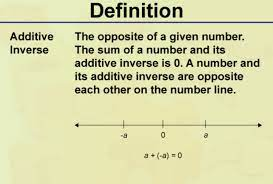d Problem18: Additive inverse of 4/3​ | Job Exam Rare Mathematics (JEMrare) - Solved MCQs

# Problem18: Additive inverse of 4/3​

Solution:

Additive inverse of a term is another term which if added to the first will give us additive identity!

Additive identity is 0So if have ‘x’ term then its additive inverse will be ‘-x’

because if we add these together i-e;

$x+(-x)=x-x=0$

$\frac{4}{3}+(-\frac{4}{3})=\frac{4}{3}-\frac{4}{3}=0$